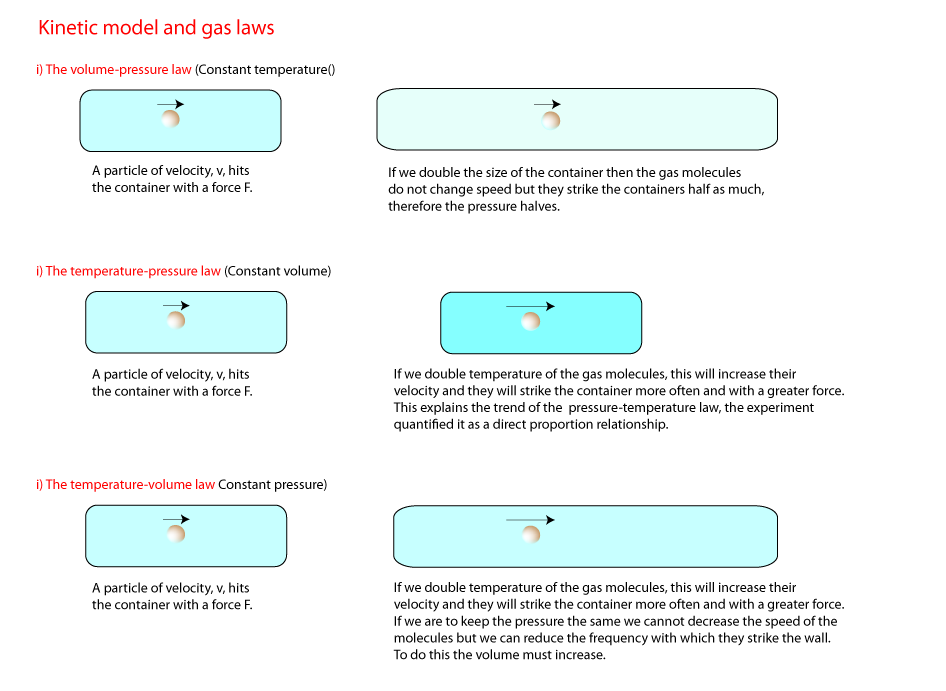Aids to Understanding

Fluids

Gases and liquids are classified as fluids because they can flow. However two gas molecules have ten times more space between than two liquid molecules and fill up whatever container they occupy. This means gases can be easilly compressed and easilly expand with rising tempertures. This opens up opportunities for investigation in gases. Namely; Volume and Pressure and Temperature and pressure.

P-V problem

Find an expression for the pressure of the combined spherical flasks when the valve is open. Suppose the temperature of the gas stays constant. The pressure in the smaller flask is zero when the valve is closed. You may ignore any gas in the joining tube.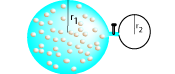Suppose the lower flask has a radius exactly one half of the upper flask. By what ratio does the pressure decrease when the valve is open?

Proportionality

If the result of an investigation yields a straight line through the origin then the quantities we investigated are in direct proportion. There are two conditions for direct proportionality.
i) Both quantities have to be zero at the same time.
ii) The quantities must change by the same factor. ie if the independent variable doubles or triples so does the dependent variable.
A direct proportion relationship is of the form Y = mx, ie V = IR or F = ma.
When we investigate temperature and pressure, when temperature is measured in degrees celcius we get a line of the form Y = mx + C. Where Y is pressure and x is temperature. Although this expression allows us to make predictions it is not a direct proportion relationship so it becomes difficult to combine the results of our gas investigations into one expression. The answer is to introduce a new temperature scale - the Kelvin scale. Each one degree change in degrees celcius corresponds to a one degree change in kelvin. Note: 0 K is equal to -273.15. This value is known as Absolute zero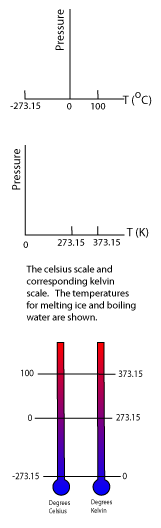P-V-T problem

This is a continuation of the above problem but now we extend it to include our knowledge of the temperature and pressure law. By what ratio must the temperature increase if the pressure is to remain what is was before the valve was open?

P-V problem

How would you use this apparatus to find the volume of a marble? Suggest possible values and try to write your own problem.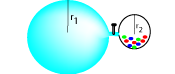Atmospheric Pressure

Although we don't notice we are a bit like fish. Where fish live in a sea or river of water we live in a sea of air. This air is colliding with us in all directions and exerting a pressure. The pressure of the air, or air pressure, tends to squash us but since we have air inside our bodies that tends to inflate us we are in equilibrium with the air outside. You may think air pressure is quite small but remember the air that extends from the earth to where it merges with outer space is about 100 km. That is a lot of air pressing down on us (even if air density decreases with distance). This air pressure has been measured to be about 100 KPa. This means air pressure can support a mass of 10, 000 kg sitting on a 1 m platform. A large elephant can weigh up to 5000 kg.The temperature - volume law

This law is sometimes called Charles Law. In this investigation we keep the pressure of the gas constant as we increase the temperature. As the gas in the tube is heated it is allowed to expand. The air pressure inside the tube always, but no more, supports the same mercury blob. Thus the pressure exerted of the gas does not change.

The general gas equation

Since P ∝1/V and V ∝T and P∝T it follows that P1V1/T1 is equal to a constant value therefore we can say that; P1V1/T1 = P2V2/T2.
This is known as the general gas equation .
You are invited to work out the units of PV/T.
Suppose a fixed mass of gas is at a Temperature, T1, Volume, V1 and Pressure P1. If the temperature decreases by a factor of 3 and the volume also decreases by a factor of 3, then what will the ratio of P1/P2 be?

The general gas equation and graphs

The graphs below summarise the results of our gas investigations. T1, V1, and P1 all indicate that the activities took place at constant temperature, volume and pressure respectively.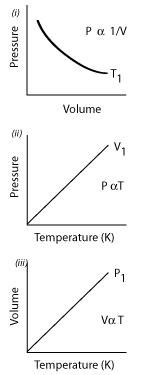You are invited to copy out these graphs and draw on them how they would change she if..
(i) The activity was completed at a temperature higher and lower than T1.
(ii)The activity was completed at a volume higher and lower than V1.
(ii)The activity was completed at a pressure higher and lower than P1.

The gas laws and kinetic model

Suppose we have a container of gas at atmospheric pressure and a temperature of 300K. (i) Suppose the volume of the container falls by one third and its temperature rises three times. Calculate the new pressure of the gas.

(ii) Explain this result using the kinetic model. A representative sample of answers will be posted here.

## 1) Kinetic model and gas pressure

The kinetic model of gases refers to gas particles in motion. In the previous topics the pressure was due to a pressing force over an area. When discussing gas pressure we think of gas particles colliding with walls and rebounding. The particle that has rebounded has undergone a change in velocity, therefore it experienced a force, a reaction force. The action force is the force the gas particle strikes the wall (or any surface) with and this force causes the pressure.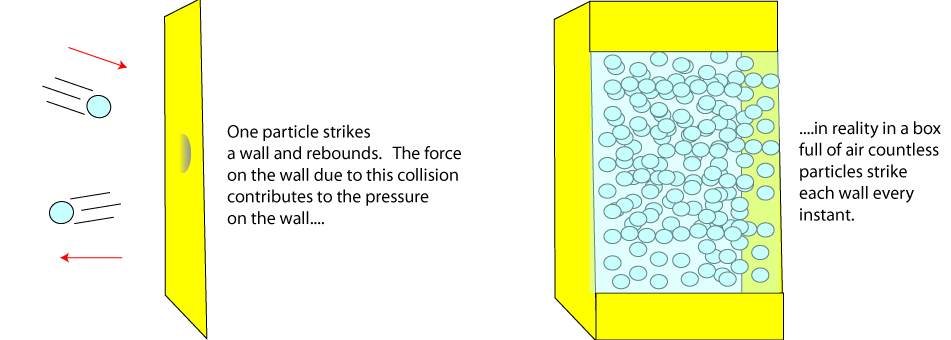## 2) Volume and pressure

The illustration below demonstrates the volume-pressure law (Sometimes called Boyle's Law). Decreasing the volume of gas increases the amount of collisions with a unit area in a a unit time (and vice versa). This means the pressure will increase and it is simple enough to see the inverse proportional nature of the volume-pressure relations. That is, if we double or half the volume we half and double the pressure respectively. The appropriate graphs are shown on the right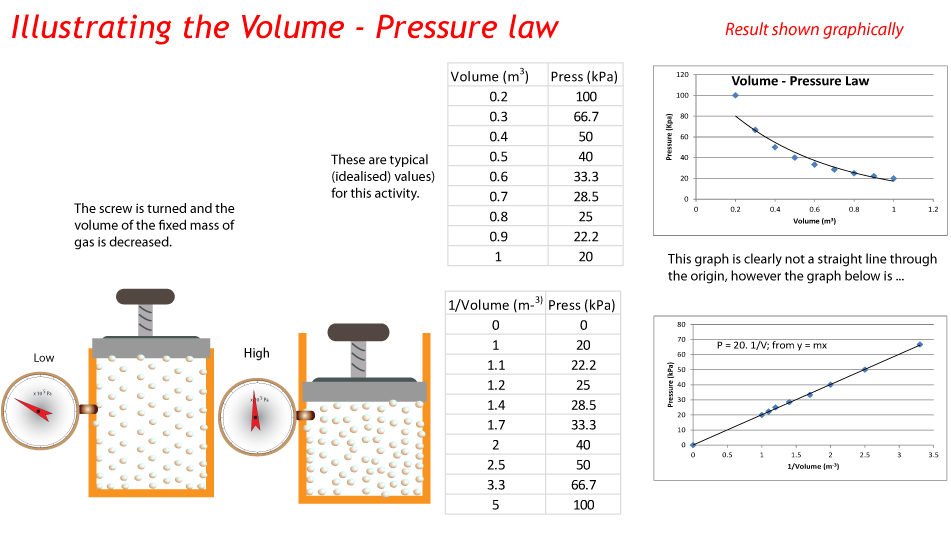The lower grapgh shoes that pressure and volume are inversely proportional or equivalently pressure is directly proportional to 1/Volume. The gradient of this line (20 units) is the same whatever pair of points you choose. ie P1V1 = P2V2.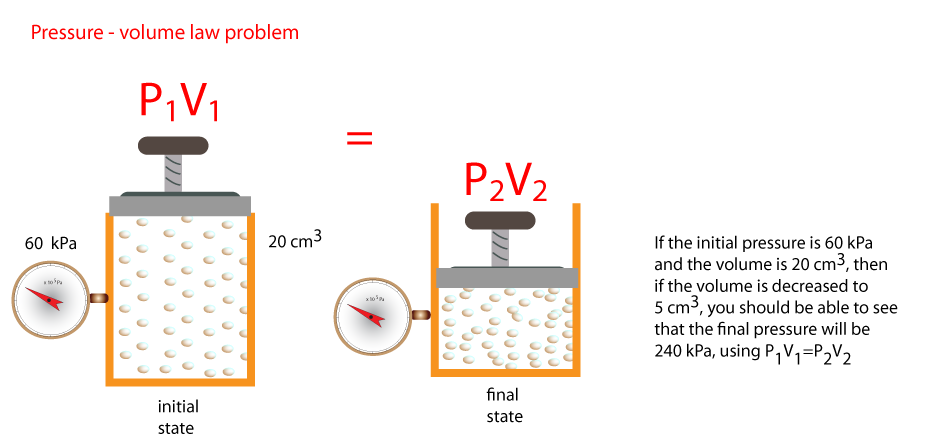The subcripts 1 and 2 indicate the initial and final states of pressure and volume. You should be able to see that if we have any three of these quantities, it is easy to find a fourth.

## 3) Temperature and pressure.

In this activity we change the temperature of a fixed mass and volume of gas and measure the pressure. we have an immediate problem though the pressure of a gas is not zero when the temperature is zero degrees centigrade. This means temperature and pressure do not vary proportionally when the temperature scale is in degrees centigrade. Zero degrees celcius is defined as the temperature water freezes or ice melts. This means, and we will see why later, we need to devise a new temperature scale that does indicate zero pressure at zero temperature. This is called the Kelvin temperature scale. Study the diagrams and graphs below to see how the Kelvin scale scale follows from the celsius scale.The line is extrapolated until it cuts the temperature line when the pressure is zero. This occurs at -273o. The blue graph has the same gradient as the first graph but, since the graph cuts the axis at point (0,0) we have a direct proportionality relationship. The lower graph shoes that temperature and pressure are directly proportional. The gradient of this line (0.35 units) is the same whatever pair of points you choose. ie P1/T1 = P2/T2.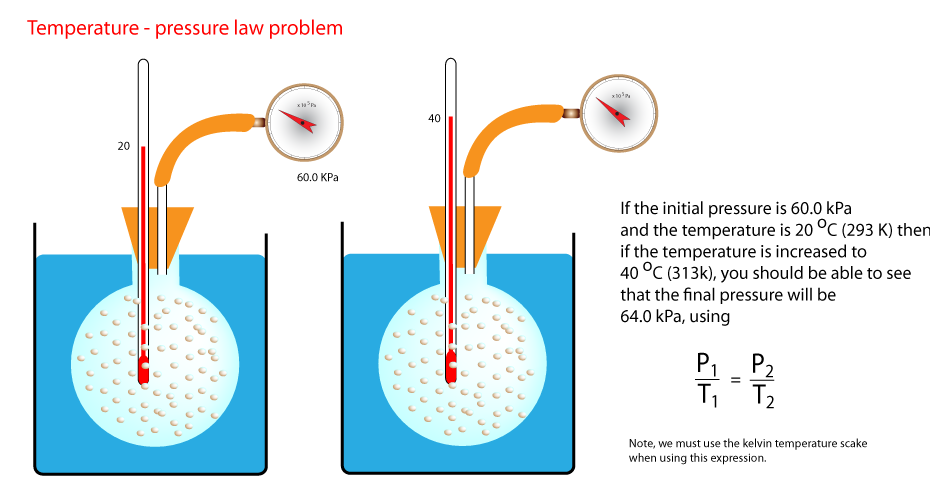## 4) Volume and temperature

In this activity we change the temperature of a fixed mass of gas at a fixed pressure and measure the volume. Look carefully at the diagram and see how the pressure of the gas is kept constant.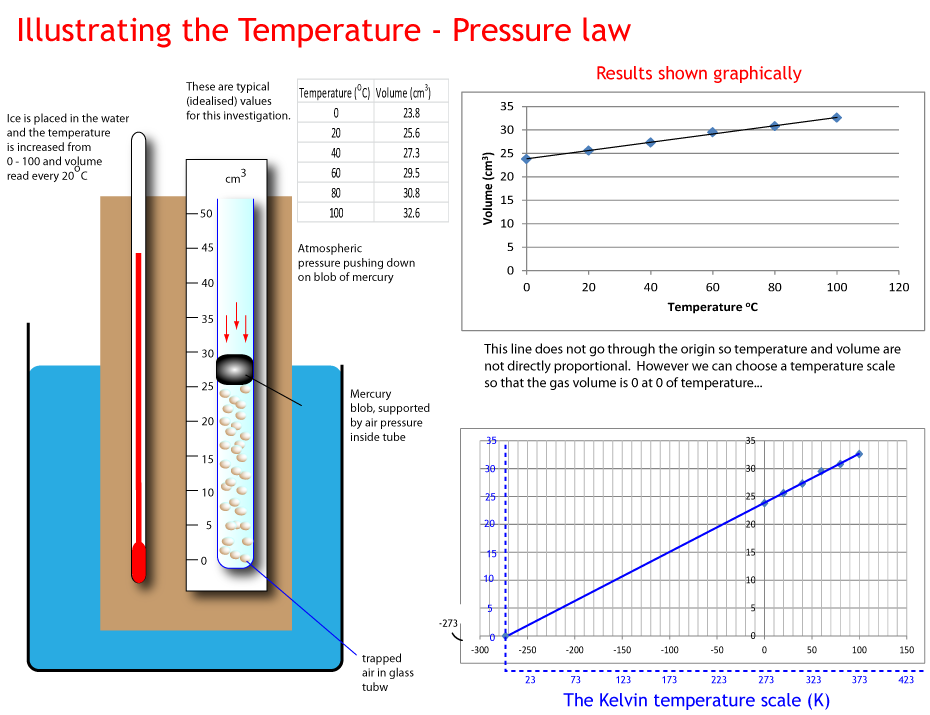The graph shoes that temperature (in Kelvin) and volume are directly proportional. The gradient of this line is the same whatever pair of points you choose. ie T1/V1 = T2/V2.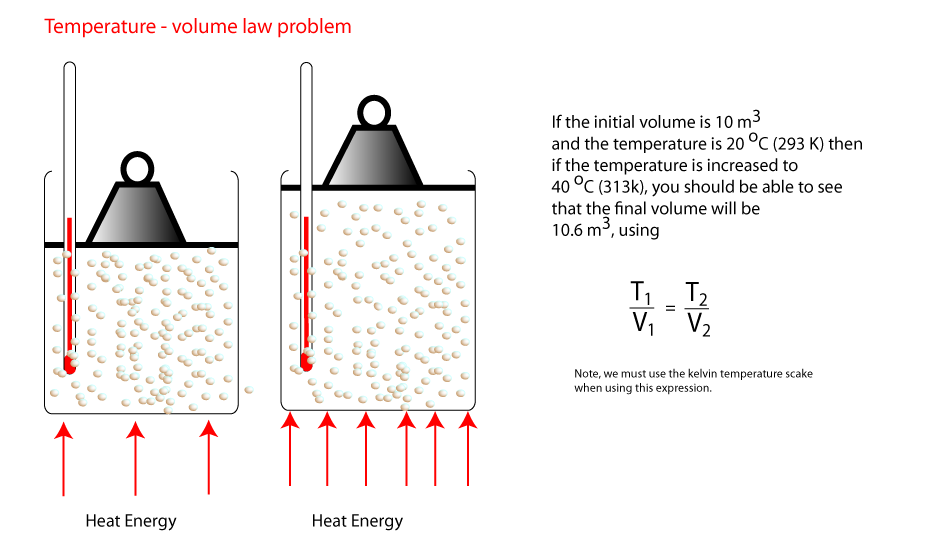## 5) Temperature scale conversions

Changing from degrees celsius to kelvin is important and for most problems you will be aked to do it will probably be necesssary. The table below has some missing values, it should be a simple matter to fill them in. Remember 0K = -273oC, so to convert from degrees celsius to Kelvin we add 273 and vice versa from converting from kelvin to degrees celsius. You are invited to fill in a third column for the fahrenheit scale.
Object, body or substance Temperature (K) Temperature (oC)
Freezing Water - 0
Boiling Water - 373
Body Temperature - 37
A nice day - 23
Absolute Zero 0 -

## 6) The general gas equation

The results of all our investigations can be gathered into one expression called the General Gas Equation. At our stage in our studies we can see that the pressure (the force with which the gas moleculues strike a unit are of its container) of a fixed mass of gas depends on the volume of its container, and the temperature of the gas.## 7) Absolute zero

If we look at the graphs of temperaure and pressure and temperature and volume, we see the temperature when the pressure of the gas and the volume it occupies is zero if the temperature in degrees celcuis in -273.15oC = 0 K. This is the point when all kinetic activity of the gas ceases. It is a hypothetical value and impossible to reach in practice, although we can get pretty close. The average temperature of the universe, (due to cosmic radiation) for example is about 2.7 K. Remember tO convert from the kelvin scale to the degrees celsuis scale we subract 273.

## 8) Gas Laws and kinetic model

It is possible to explain the gas laws using the kinetic theory. First we start with some reasonable assumptions and use them to explain the gas laws.
1. Gas particles are like tiny hard balls
2. When a gas particle strikes the surface of its container, an elastic collision occurs.
3. The pressure depends on the frequency of collisions.
4. The pressure depends on the force of the collisions
5. The force of the collisions depends on the speed of the gas molecules.
6. To increase the speed of the gas molecules we need to increase their kinetic energy, and this can only be done if we increase their temperature.